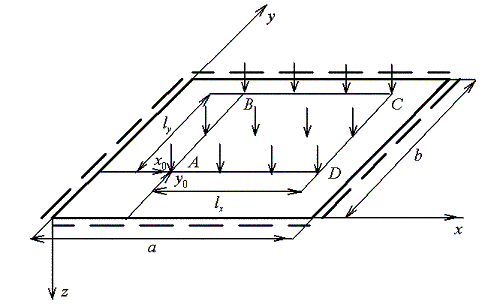Abstract General information Examples Instructions for calculation
You can run this calculation for free only for square plates, otherwise you will need to purchase a subscription

 Bending of orthotropic plateInput:

 Length of plate in x-axis direction a >0: Length of plate in y-axis direction b >0: Elasticity modulus in x-axis direction Ex >0: Elasticity modulus in y-axis direction Ey >0: Poisson's ratio of the material νyx ≥0: Shear modulus of the material G ≥0: : Plate thickness h >0: Uniform load q >0: Coordinate x of point A of load area 0≤ x0 < a: Coordinate y of point A of load area 0≤ y0 < b: Length of load area in x-axis direction 0< lx ≤ (a-x0): Length of load area in y-axis direction 0< ly ≤ (b-y0):